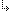- 2.2.6.12. -2. TMT Pascal Language Description2.2. Pascal Language Structure2.2.6. Types2.2.6.12. Set Types

## 2.2.6.12. Set Types

Set types specify a subset of a set of values. The ordinal value of the set elements must range between 0 and 255.
• Characters
• Enumeration type
• Positive integer values
• Subrange of the above three
• Ordinal types
Each value in a set is defined by one bit, therefore each value is similar to a Boolean. The following are examples of sets:
```type
set of 0..7;
set of '0'..'9';
set of Char;```

- 2.2.6.12. -